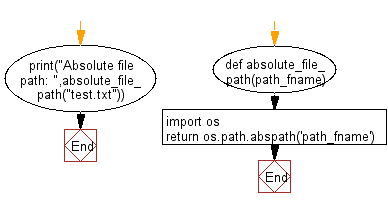﻿ Python: Get an absolute file path - w3resource

# Python: Get an absolute file path

## Python Basic: Exercise-63 with Solution

Write a Python program to get an absolute file path.

Sample Solution-1:

Python Code:

``````def absolute_file_path(path_fname):
import os
return os.path.abspath('path_fname')
print("Absolute file path: ",absolute_file_path("test.txt"))
```
```

Sample Output:

```Absolute file path:  /home/students/path_fname
```

Flowchart:## Visualize Python code execution:

The following tool visualize what the computer is doing step-by-step as it executes the said program:

Sample Solution-2:

Python Code:

``````from pathlib import Path
p = Path("main.py").resolve()
print(p)
```
```

Sample Output:

```/tmp/sessions/9f576d81597fd882/main.py
```

Python Code Editor:

Have another way to solve this solution? Contribute your code (and comments) through Disqus.

What is the difficulty level of this exercise?

Test your Programming skills with w3resource's quiz.

﻿

## Python: Tips of the Day

Decompose a collection:

Assume we have a function that returns a tuple of two values and we want to assign each value to a separate variable. One way is to use indexing as below:

```abc = (5, 10)
x = abc
y = abc
print(x, y)
```

Output:

```5 10
```

There is a better option that allows us to do the same operation in one line.

```x, y = abc
print(x, y)

```

Output:

```5 10
```

It can be extended to a tuple with more than 2 values or some other data structures such as lists or sets.

```x, y, z = {1, 2, 3}
print(x, y, z)
x, y, z = ['a', 'b', 'c']
print(x, y, z)
```

Output:

```1 2 3
a b c
```